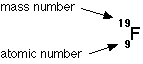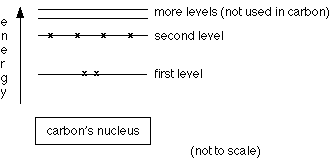# Simple View of Atomic Structure

$$\newcommand{\vecs}{\overset { \rightharpoonup} {\mathbf{#1}} }$$ $$\newcommand{\vecd}{\overset{-\!-\!\rightharpoonup}{\vphantom{a}\smash {#1}}}$$$$\newcommand{\id}{\mathrm{id}}$$ $$\newcommand{\Span}{\mathrm{span}}$$ $$\newcommand{\kernel}{\mathrm{null}\,}$$ $$\newcommand{\range}{\mathrm{range}\,}$$ $$\newcommand{\RealPart}{\mathrm{Re}}$$ $$\newcommand{\ImaginaryPart}{\mathrm{Im}}$$ $$\newcommand{\Argument}{\mathrm{Arg}}$$ $$\newcommand{\norm}{\| #1 \|}$$ $$\newcommand{\inner}{\langle #1, #2 \rangle}$$ $$\newcommand{\Span}{\mathrm{span}}$$ $$\newcommand{\id}{\mathrm{id}}$$ $$\newcommand{\Span}{\mathrm{span}}$$ $$\newcommand{\kernel}{\mathrm{null}\,}$$ $$\newcommand{\range}{\mathrm{range}\,}$$ $$\newcommand{\RealPart}{\mathrm{Re}}$$ $$\newcommand{\ImaginaryPart}{\mathrm{Im}}$$ $$\newcommand{\Argument}{\mathrm{Arg}}$$ $$\newcommand{\norm}{\| #1 \|}$$ $$\newcommand{\inner}{\langle #1, #2 \rangle}$$ $$\newcommand{\Span}{\mathrm{span}}$$$$\newcommand{\AA}{\unicode[.8,0]{x212B}}$$

## The sub-atomic particles

The table below gives relative masses and relative charges for protons, neutrons, and electrons:

Relative Mass Relative Charge
Proton 1 +1
Neutron 1 0
Electron 1/1836 -1

In reality, protons and neutrons do not have exactly the same mass; neither of them has a mass of exactly 1 on the carbon-12 scale (the scale on which the relative masses of atoms are measured). On this scale, a proton has a mass of 1.0073, and a neutron a mass of 1.0087. The masses are given as 1 for simplicity and convenience.

## Behavior of protons, neutrons and electrons in electric fields

If a beam containing each of these particles is passed between two electrically charged plates—one positive and one negative—the following are observed:

• Protons are positively charged and are thus deflected on a curving path towards the negative plate.
• Electrons are negatively charged and are deflected on a curving path towards the positive plate.
• Neutrons have no charge, and continue on in a straight line.

The exact manner in which these events occur depends on whether the particles have the same energy.

## Particles with the same energy

If beams of the three types of particles, all with the same energy, are passed between two electrically charged plates, the following is observed:

• Protons are deflected on a curved path toward the negative plate.
• Electrons are deflected on a curved path toward the positive plate.
• Neutrons continue in a straight line.

If the particles have the same energy, the magnitude of the deflection is exactly the same in the electron beam as in the proton beam, but the deflections occur in opposite directionsIf the electric field is strong enough, then the electron and proton beams could curve enough to hit their respective plates.

## If the particles have the same speed

If beams of the three particles, all with the same speed, are passed between two electrically charged plates:

• Protons are deflected on a curved path toward the negative plate.
• Electrons are deflected on a curved path toward the positive plate.
• Neutrons continue in a straight line.

If the electrons and protons travel with the same speed, then the lighter electrons are deflected far more strongly than the heavier protons.## The nucleus

The nucleus, located at the center of the atom, contains the protons and neutrons. Protons and neutrons are collectively known as nucleons. Virtually all the mass of the atom is concentrated in the nucleus because electrons weigh so little in comparison to the nucleons.

Determining numbers of protons and neutronsNumber of protons = ATOMIC NUMBER of the atom

The atomic number is also given the more descriptive name of proton number.Number of protons + number of neutrons = MASS NUMBER of the atom

The mass number is also called the nucleon number.

This information can be expressed in the following form:The atomic number is the number of protons (9); the mass number counts protons + neutrons (19). If there are 9 protons, there must be 10 neutrons adding up to a total of 19 nucleons in the atom.

The atomic number is tied to the position of the element in the periodic table; the number of protons therefore defines the element of interest. If an atom has 8 protons (atomic number = 8), it must be oxygen. If an atom has 12 protons (atomic number = 12), it must be magnesium.

Similarly, every chlorine atom (atomic number = 17) has 17 protons; every uranium atom (atomic number = 92) has 92 protons.

## Isotopes

The number of neutrons in an atom can vary within small limits. For example, there are three kinds of carbon atom: 12C, 13C and 14C. They all have the same number of protons, but the number of neutrons varies.

Protons Neutrons Mass Number
Carbon-12 6 6 12
Carbon-13 6 7 13
Carbon-14 6 8 14

Atoms with the same atomic number but different mass numbers are called isotopes. Varying numbers of neutrons nave no effect on the chemical properties of the atom.

## The electrons

Determining the number of electrons

Atoms are electrically neutral, and the positive charge from the protons is balanced by negative charge from the electrons. It follows that in a neutral atom:number of electrons = number of protons

Therefore, if an oxygen atom (atomic number = 8) has 8 protons, it must also have 8 electrons; if a chlorine atom (atomic number = 17) has 17 protons, it must also have 17 electrons.

### The arrangement of the electrons

Electrons are found at considerable distances from the nucleus, arranged in successive energy levels. Each energy level can only hold a certain number of electrons. The first level (nearest the nucleus) holds two electrons, and the second and third levels each hold eight. These levels can be visualized as getting successively further from the nucleus. Electrons always occupy the lowest possible energy level (nearest the nucleus), provided there is space.

Determining the electronic arrangement of an atom

• Look up the atomic number in the periodic table. This is the number of protons, and hence the number of electrons.
• Arrange the electrons in levels, always filling up an inner level before filling an outer one.

This process is demonstrated using chlorine:

• The periodic table gives an atomic number of 17. Therefore, there are 17 protons and 17 electrons.
• The arrangement of the electrons is 2, 8, 7 (i.e. 2 in the first level, 8 in the second, and 7 in the third).

The electronic arrangements of the first 20 elementsAfter the third level, the pattern is altered due to the transition series.

## Two Important Generalizations

Examining the patterns in the table above reveals the following:

• The number of electrons in the outermost level is the same as the group number (helium is the exception because it has only 2 electrons). This pattern extends throughout the periodic table for the main groups (excluding the transition metals and rare earth metals). Barium is in group 2, and it has 2 electrons in its outer level; iodine (group 7) has 7 electrons in its outer level; lead (group 4) has 4 electrons in its outer level.
• Noble gases have full outer levels.

### Dots-and-crosses diagrams

Introductory chemistry courses generally depict the electronic structures of hydrogen and carbon in the following way:This is a simplification and can be misleading. It gives the impression that the electrons are circling the nucleus in orbits like planets around the sun. It is impossible to know exactly how they are actually moving.

The circles show energy levels - representing increasing distances from the nucleus. If the circles are straightened, the electronic structure is shown in a simple energy diagram. The energy diagram for carbon is shown below:This visualization of the arrangement of the electrons is useful in understanding electronic structure.

This page titled Simple View of Atomic Structure is shared under a CC BY-NC 4.0 license and was authored, remixed, and/or curated by Jim Clark.